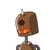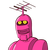# Use Euclid’s division lemma to show that the cube. of any positive integer is of the form 9m,9m+1 or 9m+8 .​

Use Euclid’s division lemma to show that the cube. of any positive integer is of the form 9m,9m+1 or 9m+8 .​

### 2 thoughts on “Use Euclid’s division lemma to show that the cube. of any positive integer is of the form 9m,9m+1 or 9m+8 .​”

1.$$\: \huge\bf\underline \red{\underline{Answer}}$$

Let a be any positive integer and b = 3

a = 3q + r, where q ≥ 0 and 0 ≤ r < 3

$$∴ r = 0,1,2$$

Therefore, every number can be represented as these three forms. There are three cases.

Case 1:

When a = 3q,

Where m is an integer such that m =

$$(3q)³ = 27q³$$

$$= > 9(3q³) = 9m$$

Case 2:

When a = 3q + 1,

$$a = (3q +1) ³$$

$$= > a = 27q ³+ 27q ² + 9q + 1$$

$$= > a = 9(3q ³ + 3q ² + q) + 1$$

$$= > a = 9m + 1$$

$$( Where \: m = 3q³ + 3q² + q )$$

Case 3:

When a = 3q + 2,

$$a = (3q +2) ³$$

$$= > a = 27q³ + 54q² + 36q + 8$$

$$= > a = 9(3q³ + 6q² + 4q) + 8$$

$$= > a = 9m + 8$$

Where m is an integer such that m =

$$(3q³ + 6q² + 4q)$$

Therefore, the cube of any positive integer is of the form 9m, 9m + 1, or 9m + 8.

2.## ❥คɴᎦᴡєя

Let us consider a and b where a be any positive number and b is equal to 3.

According to Euclid’s Division Lemma

a = bq + r

So r is an integer greater than or equal to 0 and less than 3.

Hence r can be either 0, 1 or 2.

Case 1: When r = 0, the equation becomes

a = 3q

Cubing both the sides

a3 = (3q)3

a3 = 27 q3

a3 = 9 (3q3)

a3 = 9m

where m = 3q3

Case 2: When r = 1, the equation becomes

a = 3q + 1

Cubing both the sides

a3 = (3q + 1)3

a3 = (3q)3 + 13 + 3 × 3q × 1(3q + 1)

a3 = 27q3 + 1 + 9q × (3q + 1)

a3 = 27q3 + 1 + 27q2 + 9q

a3 = 27q3 + 27q2 + 9q + 1

a3 = 9 ( 3q3 + 3q2 + q) + 1

a3 = 9m + 1

Where m = ( 3q3 + 3q2 + q)

Case 3: When r = 2, the equation becomes

a = 3q + 2

Cubing both the sides

a3 = (3q + 2)3

a3 = (3q)3 + 23 + 3 × 3q × 2 (3q + 1)

a3 = 27q3 + 8 + 54q2 + 36q

a3 = 27q3 + 54q2 + 36q + 8

a3 = 9 (3q3 + 6q2 + 4q) + 8

a3 = 9m + 8

Where m = (3q3 + 6q2 + 4q)therefore a can be any of the form 9m or 9m + 1 or, 9m + 8.